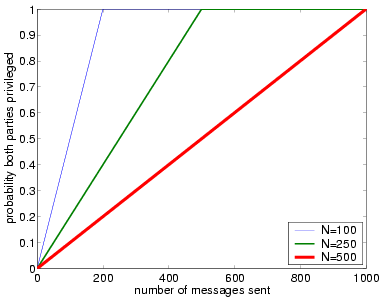# Probabilistic Fair Exchange

(Rabin)

### Contents

Related publications: [NS06]

### Introduction

This case study concerns Rabin's protocol for probabilistic fair exchange [Rab81]. Rabin's protocol enables two parties, A and B, to exchange their commitments to a pre-defined contract C with the help of a trusted third party. The protocol is not} optimistic: an action of the third party is required in every instance of the protocol. It is assumed that, every day, the third party publicly broadcasts a randomly chosen integer between 1 and N.

Before beginning the exchange, the parties agree on some future cutoff date D. A then sends to B message:

 "sigA(I am committed to C, if integer i is chosen on date D}),"

where i is initially 1. When B receives this message, he responds with

 "sigB(I am committed to C, if integer i is chosen on date D}),"

to which A responds with

 "sigA(I am committed to C, if integer i+1 is chosen on date D}),"

and so on. The exchange must complete before date D.

Intuitively, for any integer i between 1 and N, if the last message in the exchange was from B, then A and B have equal probability of being committed after the third party randomly chooses and broadcasts a number. If the number is less than or equal to i, then both parties are committed. If the number is greater than i, then neither party is committed.

Now suppose the last message in the exchange was from A. If the number broadcast by the third party is less than or equal to i, then both parties are committed. If it is greater than i+1, then neither is committed. If the number is exactly equal to i+1, then A is committed, but B is not. Therefore, the probability that the protocol terminates in a state in which only one party is committed is 1/N.

### The Model

We model the normal behaviour of protocol participants and the judge according to the protocol specification. A dishonest participant might be willing to deviate from the protocol in an attempt to cheat the honest participant. We equip the dishonest participant with an additional set of dishonest actions, any of which he can take nondeterministically at any point in the protocol. To obtain a finite probabilistic model, we fix the parameter N, that is he probability space of the judge's coin flips.

#### Modelling the dishonest participant.

Conventional formal analysis of security protocols is mainly concerned with security against the so called Dolev-Yao attacker, following [DY83]. A Dolev-Yao attacker is a nondeterministic process that has complete control over the communication network and can perform any combination of a given set of attacker operations, such as intercepting any message, splitting messages into parts, decrypting if he knows the correct decryption key, assembling fragments of messages into new messages and replaying them out of context, etc.

We assume that the digital signature scheme employed by the protocol to authenticate messages between participants is secure. Therefore, it is impossible for the misbehaving participant to forge messages from the honest participant or modify their content. We will also assume that the channels between the participants and the judge are resilient, that is, it is possible for the attacker to delay messages and schedule them out of order, but he must eventually deliver every message to its intended recipient.

Dishonest actions available to a misbehaving participant are thus limited to stopping prematurely. When combined with the probabilistic behaviour of the judge, the nondeterminism of the dishonest participant gives rise to a Markov decision process.

Following this construction process, in the case when N=10, the specification of the protocol used as input into PRISM is given by:

```// probabilistic fair exchange [Rab81]
// Gethin Norman and Vitaly Shmatikov 2004

mdp

module parties

mA : [0..10]; // number in the last message sent by A
mB : [0..10]; // number in the last message sent by B
turn : [0..1]; // who last sent a message (0- B did and 1 - A did)

// can only send messages when d=0 (i.e. before the future date arrives)
[] turn=0 & mA<10 & d=0 -> (turn'=1) & (mA'=mA+1); // party A sends a message
[] turn=1 & mA<10 & d=0 -> (turn'=0) & (mB'=mA); // party B sends a message

endmodule

module date

d : [0..1];

[] d=0 -> (d'=1); // future date arrives

endmodule

module third_party

i  : [0..10]; // integer chosen

// randomly choose integer on the future date
[] i=0 & d=1 -> 1/10 : (i'=1)
+ 1/10 : (i'=2)
+ 1/10 : (i'=3)
+ 1/10 : (i'=4)
+ 1/10 : (i'=5)
+ 1/10 : (i'=6)
+ 1/10 : (i'=7)
+ 1/10 : (i'=8)
+ 1/10 : (i'=9)
+ 1/10 : (i'=10);

endmodule
```

Note that there is a nondeterministic choice as to when the date is reached and the sending of messages which models the fact that a party can stop sending messages at any time.

### Analysis Results

In Rabin's protocol, unfair states are defined by the condition

(i>0) & (mA>=i) & (mB<i)

that is, the number i randomly chosen by the third party is such that B possesses A's commitment, but A does not possess B's commitment.

We have computed the maximum probability of reaching such a state, that is verified the formula:

Pmax=?[true U (i>0) & (mess1>=i) & (mess2<i) ]

for a number of different values of N (the results of which are given in the table below) and, as expected, in each case the maximum probability computed equals 1/N.

 N: Number of states: Result: 10 240 0.1 50 5,200 0.02 100 20,400 0.01 250 126,000 0.004 500 502,000 0.002 750 1,128,000 0.0013 1,000 2,004,000 0.001

The probability of reaching a state in which both parties are committed as a function of the number of message rounds is plotted below for different values of N. This figure illustrates that probabilistic fairness depends linearly on the number of rounds in Rabin's protocol.